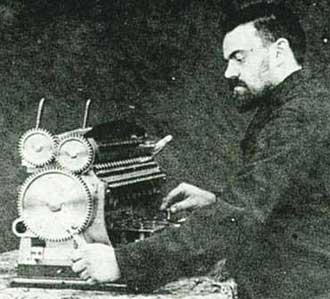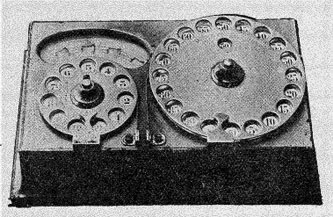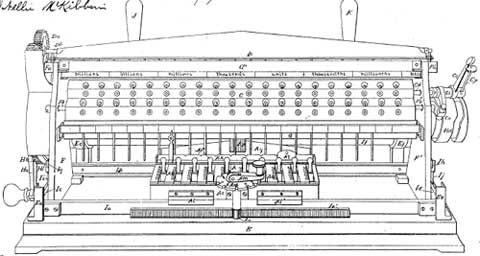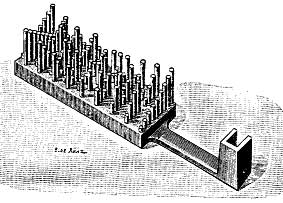### León Bollée

After the first two machines, representing the class of direct-multiplying machines of Barbour and Verea, which remained relatively unknown, appeared a third one, which had a much better future—the machine of León Bollée (see biography of León Bollée) of Le Mans, France, which won a gold medal at the 1889 Paris Exposition.

Most early calculating machines carried out multiplication as a form of repeated addition. To multiply, say, by sixteen, one set the carriage at its rightmost position, turned the operating crank six times, shifted the carriage one position to the left, and turned the crank once. In direct-multiplying calculating machines, the operator had only to perform n operations when the multiplier was an n digit number.Léon Bollée (1870-1913) was a famous French inventor, auto manufacturer and racing enthusiast. Besides his passion to the automobiles, quite young Léon Bollée was obcessed by another passion—calculating machines and devices. Starting from 1887, he devised several calculating machines: Direct Multiplier, Arithmographe (Small Multiplicator), Calculating Board, Small Adding Machine (see the nearby image), etc.

The Arithmographe of Bollée is a simple mechanical calculating tool for multiplication and division, which used rulers of Genaille-Lucas, arranged in sheets (see the photo below).The Arithmographe of Bollée from 1889 (©INRIA, photo J.-M. Ramès, collection IBM-Europe)

The Multiplier of Bollée was actually the first successful direct-multiplying calculator and it won a gold medal at the 1889 Paris Exposition (1889 Exposition universelle in Paris). The devices (Multiplier and Arithmographe) were patented in France (1889, No FR201033), Belgium, Germany (DE88936 and DE82963), Austria-Hungary, England (GB16677) and USA (patent No 556720 from 1896) (see the figure below).

It was a large manually operated non-printing direct multiplication calculating machine has an iron base with a brass and steel mechanism and an open framework.A patent drawing of the calculating machine of Bollée

The main advantage of all direct-multypling machines over the other calculating machines of this time, is the speed of calculations. In 1892, during a demonstration, Bollée calculate automatically the square root of an 18 digit number in about 30 seconds, a remarkable speed for this time. Similar calculation with other machine would require at least 5 minutes.The multiplying calculating machine of Bollée (Courtesy Nico Baaijens, www.calculi.nl)

The idea, used in the multiplying calculating machine of Bollée is to use special bars with attached pins with different length, to be created something like a mechanical representation of the Napier's bones. The different possible products are presented by means of two plates: first is for units (see the lower sketch), second is for tens. The height of the pins is proportional to the digit, which is in this particular position of the product.

A metal box carrying 12 setting levers moves along a bar near the front of machine. In front of it are a multiplier knob and brass disc with 20 teeth. The spaces between the teeth are labeled from 0 to 9 and from 0 to 9 again around the edge. Rotating the multiplier knob moves the metal box, placing it in one of the spaces between the teeth of the disc sets the digit of the multiplier.

The bar with pins is shifted in such manner, that during the transfer of the number from the setting mechanism to the calculating mechanism the pins will come into contact with gear-strips and will move them to a different distance, according to the height of the particular pin. So the wheels of the counter, which will be engaged to this gear-strips will be rotated to the different angle and thus the right digit will be transferred. So all the multiplication will be done with one movement of the calculating mechanism, not by consecutive addition or subtraction, as in other used for multiplication and division machines.The bar for units from multiplying machine of Bollée

On the right side is a lever that may be set at either + or -. Toward the front is a set of 21 cylindrical dials, visible through windows. Each dial shows two digits at any one time. The top row of digits is labeled MULTN and used to show the multiplier in multiplication. The lower row of digits is labeled QUOTT and shows the quotient in division. Above this row of cylinders is a row of 21 dials which shows the result in multiplication and is set with thumbscrews with the divisor in division.

After the invention of his remarkable calculating machine Bollée turned his efforts to building cars and organizing races. He founded the company Léon Bollée Automobiles in 1895 in Le Mans. Now Bollée is known primarily as the founder of the world known car race Le Mans 24 hours.